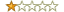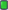#1
 dosprogFriendJoin Date: Feb 2018 Posts: 100 Rept. Given: 0 Rept. Rcvd 14 Times in 13 Posts Thanks Given: 31 Thanks Rcvd at 138 Times in 70 Postsscanfp

scanfp (c).SVD.,2001,2021

- simple command line tool for converting floating point numbers between BIN<->STR representation.

This program converts the entered floating point number into its binary representation as "DB" assembly code.

And vice versa - the binary representation of a number from a file is output as its "DD / DQ / DT" representation as an assembler string.

The program works with three types of floating point numbers
- DD - float (32bit)
- DQ - double (64bit)
- DT - long double (80bit FPU)

This program works in pure DOS and in WIN_ALL (DUAL WIN32 PE-EXE).

DOS16-stub created 2001 using TurboC v.2.0
WIN32-cmd crated 2021 using BorlandC v.5.1.

Code:
```Usage: C>scanfp [f|d|l]   <input  >output
where:
f = scanf("%e" ,...) - float  32bit
d = scanf("%le",...) - double 64bit
l = scanf("%Le",...) - fpu    80bit - default!

or:    C>scanfp p [f|d|l] inpFile
where:
f = printf("%e" ,...) - float  32bit
d = printf("%le",...) - double 64bit
l = printf("%Le",...) - fpu    80bit - default!```
For example:
Code:
```String to DB code ->

C> scanfp
C> scanfp f
C> scanfp d

BINfile to DD/DQ/DT+DB code ->

C> scanfp p InputFile
C> scanfp p f InputFile
C> scanfp p d InputFile```

Sample of output:

Code:
```C> scanfp  <stdin

db 000h,000h,000h,0A0h,06Fh,0B5h,0A4h,0B3h,02Ah,040h ;;=+1.23450000000000000000e+13

C> scanfp d  <stdin

db 0EBh,0E8h,0EBh,009h,073h,02Bh,0A0h,03Ch ;;=+1.12200000000000004e-16

C> scanfp f  <stdin

db 07Dh,0FEh,0B5h,021h ;;=+1.2332400e-18

C> scanfp p 80

dt +1.20000000000000000000e-81  ;;=db 095h,041h,0F4h,099h,023h,03Eh,0B3h,091h,0F2h,03Eh

C> scanfp p d 64

dq +1.19999999999999995e-81     ;;=db 088h,03Eh,073h,0C4h,067h,036h,022h,02Fh

C> scanfp p f 32

dd +1.2000000e-08               ;;=db 08Fh,028h,04Eh,032h```